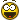# Merge duplicate words

Hi,

I would like to find in genre duplicate words and merge them together.

This is an example of genre tag:

Before

``````AFRICA; AFRICA; NATURAL WORLD; NATURE STUDY; NATURE; DRAMA ADVENTURE; DRAMA WORLD; PERCUSSION; INSTRUMENTS SOLO PERCUSSION; WILDLIFE MOVEMENT; DRAMA ADVENTURE; AFRICA; FILM STYLES ANCIENT ROME; AFRICA
``````

After

``````AFRICA;  NATURAL WORLD; NATURE STUDY; NATURE; DRAMA WORLD; PERCUSSION; INSTRUMENTS SOLO PERCUSSION; WILDLIFE MOVEMENT; DRAMA ADVENTURE;  FILM STYLES ANCIENT ROME
``````

; is the separator here
[A-Z ]*; I think will match every entry for this songs genre

AFRICA is for instance 4 times repeated and I would like to merge them into 1 time

Is there a way to do this?

Kind regards

Search there ...

Doppelte Genre löschen

DD.20141103.1721.CET

You want to keep the first value. That's more complicated.

[Edit:]
Action: Format value
Field: Genre
Format string: \$regexp(\$reverse(\$regexp(\$regexp(\$reverse(\$regexp(\$regexp(%Genre%,'^(\s+;|\s;+)+|(\s+;|\s;+)+\$|((?<=;)\s+)|(\s+(?=;))',),'^|\$',';')),'(;[^;]+)(?=(\1|;.*?\1))',),'^;+|;+\$',)),'(?<=;)',' ')

Replace Genre with any other tag and it will remove duplicates too.
This action "fixes" first the multiple value string (remove multiple spaces after ; separator).
It will add exactly one space after value separator [;].

Enjoy.Your type of answer will motivate me to search in the next time even better, if I can find it I'm helped immediatelyThank you for your patience with me

Hi,

Thank you very much,
I have tried it and it works like a charm..
Indeed I will be using it for mood tag to.

This one could definitely be in the list of examples of reg expressions.

Hartelijk bedankt
Guy ForssmanHi There,

On some longer metadata fields I get this error REGEXP ERROR: Regular expression

``````The complexity of matching the regular expression exceeded predefined bounds.  Try refactoring the regular expression to make each choice made by the state machine unambiguous.  This exception is thrown to prevent "eternal" matches that take an indefinite period time to locate.
``````

I found a workaround by eliminating excessive ; signs

Thanks again

QUOTE (ForSSOund @ Nov 28 2014, 11:21) <{POST_SNAPBACK}>
Hi There,

On some longer metadata fields I get this error REGEXP ERROR: Regular expression

``````The complexity of matching the regular expression exceeded predefined bounds.  Try refactoring the regular expression to make each choice made by the state machine unambiguous.  This exception is thrown to prevent "eternal" matches that take an indefinite period time to locate.
``````

I found a workaround by eliminating excessive ; signs

Thanks again

Simplified version.
\$reverse(\$regexp(\$regexp(';'\$reverse(%Genre%),'\s*(;[^;]+)(?=(\s*\1|;.?\1))',),'^\s;+|;+\s*\$',))

Hello! I tried to use this to do the same exact thing, but with " / " as a separator. Ex: World Music / Latin Jazz / Cuban

But it did not work. Hopefully someone can help me figure out why and how I can modify it to suit my needs.

I tried turning
\$reverse(\$regexp(\$regexp(';'\$reverse(%Genre%),'\s*(;[^;]+)(?=(\s*\1|;.?\1))',),'^\s;+|;+\s*\$',))

to

\$reverse(\$regexp(\$regexp(‘ / ‘\$reverse(%Genre%),'\s*(;[^;]+)(?=(\s*\1|;.?\1))',),'^\s;+|;+\s*\$',))
By replacing ';' with ' / ' but that resulted in
"World Music / World Music / Latin / Latin Jazz / Latin"
turning to
"World Music / World Music / Latin / Latin Jazz / Latin‘ / ‘"

I also tried using the longer version turning

\$regexp(\$reverse(\$regexp(\$regexp(\$reverse(\$regexp(\$regexp(%Genre%,'^(\s+;|\s;+)+|(\s+;|\s;+)+\$|((?<=;)\s+)|(\s+(?=;))',),'^|\$',';')),'(;[^;]+)(?=(\1|;.?\1))',),'^;+|;+\$',)),'(?<=;)',' ')
to
\$regexp(\$reverse(\$regexp(\$regexp(\$reverse(\$regexp(\$regexp(%Genre%,'^(\s+;|\s;+)+|(\s+;|\s;+)+\$|((?<=;)\s+)|(\s+(?=;))',),'^|\$',’ / ‘)),'(;[^;]+)(?=(\1|;.
?\1))',),'^;+|;+\$',)),'(?<=;)',' ')
using the same method (Using replace). But that also did not work. It turned
"World Music / World Music / Latin / Latin Jazz / " to
"' / ‘World Music / World Music / Latin / Latin Jazz / Latin’ / ‘"

It looks a lot like the forum formatter swallowed a couple important `*`.
I don't know, though, where.
As a suggestion:
you could write an action group that first replaces the slashes in your genre field with the semicolon,
then an action to process the field with the existing expression, so that you don't have to worry about replacing the semicolon with the slash inside the expression,
then, after rearranging the individual names an action that replaces the semicolons with the slash again.

Try this one from the other thread: (I adapted it for "/" as separator)

``\$trim(\$regexp(%Genre%,'(?:(?<=/)|(?<=\A))\s*([^/]*)\s*/(?=.*?(?<=/)\s*\1(?=/|\Z))',,1))``

Try this one from the other thread: (I adapted it for "/" as separator)

Thank you so much dano! I am not sure how it works but it does and this is a game changer for me.

Very grateful for your help. Hope it comes back around for you!

1 Like

Hey dano, if you could point me to the other thread, I would appreciate it as I cannot seem to find it.

Also if you be so kind as to bold where I would change the "/" to any other separator, in case I have to modify this in the future.

I assume I would just have to change the characters in bold below from "/" to any other separator I want, but I am not sure.
\$trim(\$regexp(%Genre%,'(?:(?<=/)|(?<=\A))\s*([^/])\s/(?=.?(?<=/)\s\1(?=/|\Z))',,1))

I am not sure if the "/" after the "\s*" would need to be changed as well.

So, would
\$trim(\$regexp(%Genre%,'(?:(?<=; )|(?<=\A))\s*([^; ])\s/(?=.?(?<=; )\s\1(?=; |\Z))',,1))

if I used "; " as a separator? Or would it have to be

\$trim(\$regexp(%Genre%,'(?:(?<=; )|(?<=\A))\s*([^; ])\s; (?=.?(?<=; )\s\1(?=; |\Z))',,1))

for some reason, neither will work when I switch from " / " to "; " as separators.

In fact, I even tried vkostas' simplified version with "; " as a separator and that did not work as well. Not sure why.

You need to replace all occurrences of "/"

I thought I did, but it does not seem to work.

\$trim(\$regexp(%Genre%,'(?:(?<=; )|(?<=\A))\s*([^; ])\s; (?=.?(?<=; )\s\1(?=; |\Z))',,1))

You've put a space behind each ;

Thanks. I got that cleaned up now. Much appreciated!

This topic was automatically closed 30 days after the last reply. New replies are no longer allowed.